# Attention¶

## Attention¶

class deep_qa.layers.attention.attention.Attention(similarity_function: typing.Dict[str, typing.Any] = None, normalize: bool = True, **kwargs)[source]

Bases: deep_qa.layers.masked_layer.MaskedLayer

This Layer takes two inputs: a vector and a matrix. We compute the similarity between the vector and each row in the matrix, and then (optionally) perform a softmax over rows using those computed similarities. We handle masking properly for masked rows in the matrix, though we ignore any masking on the vector.

By default similarity is computed with a dot product, but you can alternatively use a parameterized similarity function if you wish.

Inputs:

• vector: shape (batch_size, embedding_dim), mask is ignored if provided
• matrix: shape (batch_size, num_rows, embedding_dim), with mask (batch_size, num_rows)

Output:

• attention: shape (batch_size, num_rows). If normalize is True, we return no mask, as we’ve already applied it (masked input rows have value 0 in the output). If normalize is False, we return the matrix mask, if there was one.
Parameters: similarity_function_params : Dict[str, Any], optional (default: {}) These parameters get passed to a similarity function (see deep_qa.tensors.similarity_functions for more info on what’s acceptable). The default similarity function with no parameters is a simple dot product. normalize : bool, optional (default: True) If true, we normalize the computed similarities with a softmax, to return a probability distribution for your attention. If false, this is just computing a similarity score.
build(input_shape)[source]

Creates the layer weights.

Must be implemented on all layers that have weights.

# Arguments
input_shape: Keras tensor (future input to layer)
or list/tuple of Keras tensors to reference for weight shape computations.
compute_mask(inputs, mask=None)[source]

# Arguments
inputs: Tensor or list of tensors. mask: Tensor or list of tensors.
# Returns
None or a tensor (or list of tensors,
one per output tensor of the layer).
compute_output_shape(input_shapes)[source]

Computes the output shape of the layer.

Assumes that the layer will be built to match that input shape provided.

# Arguments
input_shape: Shape tuple (tuple of integers)
or list of shape tuples (one per output tensor of the layer). Shape tuples can include None for free dimensions, instead of an integer.
# Returns
An input shape tuple.
get_config()[source]

Returns the config of the layer.

A layer config is a Python dictionary (serializable) containing the configuration of a layer. The same layer can be reinstantiated later (without its trained weights) from this configuration.

The config of a layer does not include connectivity information, nor the layer class name. These are handled by Container (one layer of abstraction above).

# Returns
Python dictionary.

## GatedAttention¶

class deep_qa.layers.attention.gated_attention.GatedAttention(gating_function='*', **kwargs)[source]

Bases: deep_qa.layers.masked_layer.MaskedLayer

This layer implements the majority of the Gated Attention module described in “Gated-Attention Readers for Text Comprehension” by Dhingra et. al 2016.

The module is described in section 3.2.2. For each token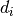in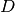, the GA module forms a “token-specific representation” of the query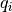using soft attention, and then multiplies the query representation element-wise with the document token representation.

1.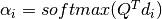1.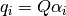1.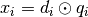(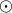is element-wise multiplication)

This layer implements equations 2 and 3 above but in a batched manner to get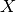, a tensor with all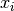. Thus, the input to the layer is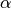(normalized_qd_attention), a tensor with all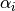, as well as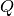(question_matrix), and(document_matrix), a tensor with all. Equation 6 uses element-wise multiplication to model the interactions betweenand, and the paper reports results when using other such gating functions like sum or concatenation.

Inputs:
• document_, a matrix of shape (batch, document length, biGRU hidden length). Represents the document as encoded by the biGRU.
• question_matrix, a matrix of shape (batch, question length, biGRU hidden length). Represents the question as encoded by the biGRU.
• normalized_qd_attention, the soft attention over the document and question. Matrix of shape (batch, document length, question length).
Output:
• X, a tensor of shape (batch, document length, biGRU hidden length) if the gating function is * or +, or (batch, document length, biGRU hidden length * 2) if the gating function is || This serves as a representation of each token in the document.
Parameters: gating_function : string, default=”*” The gating function to use for modeling the interactions between the document and query token. Supported gating functions are "*" for elementwise multiplication, "+" for elementwise addition, and "||" for concatenation.

Notes

To find out how we calculated equation 1, see the GatedAttentionReader model (roughly, a masked_batch_dot and a masked_softmax)

compute_mask(inputs, mask=None)[source]

# Arguments
inputs: Tensor or list of tensors. mask: Tensor or list of tensors.
# Returns
None or a tensor (or list of tensors,
one per output tensor of the layer).
compute_output_shape(input_shapes)[source]

Computes the output shape of the layer.

Assumes that the layer will be built to match that input shape provided.

# Arguments
input_shape: Shape tuple (tuple of integers)
or list of shape tuples (one per output tensor of the layer). Shape tuples can include None for free dimensions, instead of an integer.
# Returns
An input shape tuple.
get_config()[source]

Returns the config of the layer.

A layer config is a Python dictionary (serializable) containing the configuration of a layer. The same layer can be reinstantiated later (without its trained weights) from this configuration.

The config of a layer does not include connectivity information, nor the layer class name. These are handled by Container (one layer of abstraction above).

# Returns
Python dictionary.

class deep_qa.layers.attention.masked_softmax.MaskedSoftmax(**kwargs)[source]

Bases: deep_qa.layers.masked_layer.MaskedLayer

This Layer performs a masked softmax. This could just be a Lambda layer that calls our tensors.masked_softmax function, except that Lambda layers do not properly handle masked input.

The expected input to this layer is a tensor of shape (batch_size, num_options), with a mask of the same shape. We also accept an input tensor of shape (batch_size, num_options, 1), which we will squeeze to be (batch_size, num_options) (though the mask must still be (batch_size, num_options)).

While we give the expected input as having two modes, we also accept higher-order tensors. In those cases, we’ll first perform a last_dim_flatten on both the input and the mask, so that we always do the softmax over a single dimension (the last one).

We give no output mask, as we expect this to only be used at the end of the model, to get a final probability distribution over class labels (and it’s a softmax, so you’ll have zeros in the tensor itself; do you really still need a mask?). If you need this to propagate the mask for whatever reason, it would be pretty easy to change it to optionally do so - submit a PR.

compute_mask(inputs, mask=None)[source]

# Arguments
inputs: Tensor or list of tensors. mask: Tensor or list of tensors.
# Returns
None or a tensor (or list of tensors,
one per output tensor of the layer).
compute_output_shape(input_shape)[source]

Computes the output shape of the layer.

Assumes that the layer will be built to match that input shape provided.

# Arguments
input_shape: Shape tuple (tuple of integers)
or list of shape tuples (one per output tensor of the layer). Shape tuples can include None for free dimensions, instead of an integer.
# Returns
An input shape tuple.

## MatrixAttention¶

class deep_qa.layers.attention.matrix_attention.MatrixAttention(similarity_function: typing.Dict[str, typing.Any] = None, **kwargs)[source]

Bases: deep_qa.layers.masked_layer.MaskedLayer

This Layer takes two matrices as input and returns a matrix of attentions.

We compute the similarity between each row in each matrix and return unnormalized similarity scores. We don’t worry about zeroing out any masked values, because we propagate a correct mask.

By default similarity is computed with a dot product, but you can alternatively use a parameterized similarity function if you wish.

This is largely similar to using TimeDistributed(Attention), except the result is unnormalized, and we return a mask, so you can do a masked normalization with the result. You should use this instead of TimeDistributed(Attention) if you want to compute multiple normalizations of the attention matrix.

Input:
• matrix_1: (batch_size, num_rows_1, embedding_dim), with mask (batch_size, num_rows_1)
• matrix_2: (batch_size, num_rows_2, embedding_dim), with mask (batch_size, num_rows_2)
Output:
• (batch_size, num_rows_1, num_rows_2), with mask of same shape
Parameters: similarity_function_params: Dict[str, Any], default={} These parameters get passed to a similarity function (see deep_qa.tensors.similarity_functions for more info on what’s acceptable). The default similarity function with no parameters is a simple dot product.
build(input_shape)[source]

Creates the layer weights.

Must be implemented on all layers that have weights.

# Arguments
input_shape: Keras tensor (future input to layer)
or list/tuple of Keras tensors to reference for weight shape computations.
compute_mask(inputs, mask=None)[source]

# Arguments
inputs: Tensor or list of tensors. mask: Tensor or list of tensors.
# Returns
None or a tensor (or list of tensors,
one per output tensor of the layer).
compute_output_shape(input_shape)[source]

Computes the output shape of the layer.

Assumes that the layer will be built to match that input shape provided.

# Arguments
input_shape: Shape tuple (tuple of integers)
or list of shape tuples (one per output tensor of the layer). Shape tuples can include None for free dimensions, instead of an integer.
# Returns
An input shape tuple.
get_config()[source]

Returns the config of the layer.

A layer config is a Python dictionary (serializable) containing the configuration of a layer. The same layer can be reinstantiated later (without its trained weights) from this configuration.

The config of a layer does not include connectivity information, nor the layer class name. These are handled by Container (one layer of abstraction above).

# Returns
Python dictionary.

## MaxSimilaritySoftmax¶

class deep_qa.layers.attention.max_similarity_softmax.MaxSimilaritySoftmax(knowledge_axis, max_knowledge_length, **kwargs)[source]

Bases: deep_qa.layers.masked_layer.MaskedLayer

This layer takes encoded questions and knowledge in a multiple choice setting and computes the similarity between each of the question embeddings and the background knowledge, and returns a softmax over the options.

Inputs:

• encoded_questions (batch_size, num_options, encoding_dim)
• encoded_knowledge (batch_size, num_options, knowledge_length, encoding_dim)

Output:

• option_probabilities (batch_size, num_options)

This is a pretty niche layer that does a very specific computation. We only made it its own class instead of a Lambda layer so that we could handle masking correctly, which Lambda does not.

compute_mask(inputs, mask=None)[source]

# Arguments
inputs: Tensor or list of tensors. mask: Tensor or list of tensors.
# Returns
None or a tensor (or list of tensors,
one per output tensor of the layer).
compute_output_shape(input_shapes)[source]

Computes the output shape of the layer.

Assumes that the layer will be built to match that input shape provided.

# Arguments
input_shape: Shape tuple (tuple of integers)
or list of shape tuples (one per output tensor of the layer). Shape tuples can include None for free dimensions, instead of an integer.
# Returns
An input shape tuple.

## WeightedSum¶

class deep_qa.layers.attention.weighted_sum.WeightedSum(use_masking: bool = True, **kwargs)[source]

Bases: deep_qa.layers.masked_layer.MaskedLayer

This Layer takes a matrix of vectors and a vector of row weights, and returns a weighted sum of the vectors. You might use this to get some aggregate sentence representation after computing an attention over the sentence, for example.

Inputs:

• matrix: (batch_size, num_rows, embedding_dim), with mask (batch_size, num_rows)
• vector: (batch_size, num_rows), mask is ignored

Outputs:

• A weighted sum of the rows in the matrix, with shape (batch_size, embedding_dim), with mask=None.
Parameters: use_masking: bool, default=True If true, we will apply the input mask to the matrix before doing the weighted sum. If you’ve computed your vector weights with masking, so that masked entries are 0, this is unnecessary, and you can set this parameter to False to avoid an expensive computation.

Notes

You probably should have used a mask when you computed your attention weights, so any row that’s masked in the matrix should already be 0 in the attention vector. But just in case you didn’t, we’ll handle a mask on the matrix here too. If you know that you did masking right on the attention, you can optionally remove the mask computation here, which will save you a bit of time and memory.

While the above spec shows inputs with 3 and 2 modes, we also allow inputs of any order; we always sum over the second-to-last dimension of the “matrix”, weighted by the last dimension of the “vector”. Higher-order tensors get complicated for matching things, though, so there is a hard constraint: all dimensions in the “matrix” before the final embedding must be matched in the “vector”.

For example, say I have a “matrix” with dimensions (batch_size, num_queries, num_words, embedding_dim), representing some kind of embedding or encoding of several multi-word queries. My attention “vector” must then have at least those dimensions, and could have more. So I could have an attention over words per query, with shape (batch_size, num_queries, num_words), or I could have an attention over query words for every document in some list, with shape (batch_size, num_documents, num_queries, num_words). Both of these cases are fine. In the first case, the returned tensor will have shape (batch_size, num_queries, embedding_dim), and in the second case, it will have shape (batch_size, num_documents, num_queries, embedding_dim). But you can’t have an attention “vector” that does not include all of the queries, so shape (batch_size, num_words) is not allowed - you haven’t specified how to handle that dimension in the “matrix”, so we can’t do anything with this input.

compute_mask(inputs, mask=None)[source]

# Arguments
inputs: Tensor or list of tensors. mask: Tensor or list of tensors.
# Returns
None or a tensor (or list of tensors,
one per output tensor of the layer).
compute_output_shape(input_shapes)[source]

Computes the output shape of the layer.

Assumes that the layer will be built to match that input shape provided.

# Arguments
input_shape: Shape tuple (tuple of integers)
or list of shape tuples (one per output tensor of the layer). Shape tuples can include None for free dimensions, instead of an integer.
# Returns
An input shape tuple.
get_config()[source]

Returns the config of the layer.

A layer config is a Python dictionary (serializable) containing the configuration of a layer. The same layer can be reinstantiated later (without its trained weights) from this configuration.

The config of a layer does not include connectivity information, nor the layer class name. These are handled by Container (one layer of abstraction above).

# Returns
Python dictionary.